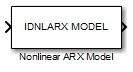Nonlinear ARX Model

Simulate nonlinear ARX model in Simulink software

• Library:
• System Identification Toolbox / Models

•Description

The Nonlinear ARX Model block simulates the output of a nonlinear ARX model using time-domain input data. The model is an idnlarx model that you previously estimated or constructed in the MATLAB® workspace. You specify initial conditions for the simulation as either steady-state input and output signal levels or as an initial state vector.

Ports

Input

expand all

Simulation input data, specified as a scalar for a single-input model. The data must be time-domain data. For multi-input models, specify the input as an Nu-element vector, where Nu is the number of inputs. For example, you can use a Vector Concatenate (Simulink) block to concatenate scalar signals into a vector signal.

Note

Do not use a Bus Creator (Simulink) or Mux (Simulink) block to produce the vector signal.

Data Types: double

Output

expand all

Simulated output from nonlinear ARX model, returned as a scalar for a single-output model and an Ny-element vector for a model with Ny outputs.

Data Types: double

Parameters

expand all

Nonlinear ARX model to be simulated, specified as an idnlarx object. You previously estimate or construct the idnlarx model in the MATLAB workspace.

The states of a nonlinear ARX model correspond to the dynamic elements of the nonlinear ARX model structure. The dynamic elements are the model regressors. Regressors can be the delayed input or output variables (standard regressors) or user-defined transformations of delayed input-output variables (custom regressors). For more information about the states of a nonlinear ARX model, see the idnlarx reference page.

For simulating nonlinear ARX models, you can specify the initial conditions one of the following:

• Input and output values — Specify steady-state input and output signal levels in Input level and Output level, respectively.

• State values — Specify a vector of length equal to the number of states in the model in Specify initial states as a vector.

Steady-state input signal level before simulation, specified as a scalar.

Dependency

To enable this parameter, specify Initial conditions as Input and output values.

Steady-state output signal level before simulation, specified as a scalar.

Dependency

To enable this parameter, specify Initial conditions as Input and output values.

Initial state values of the model, specified as an Nx-element vector, where Nx is the number of states of the model. This parameter is named Vector of state values until you specify Model.

If you do not know the initial states, you can estimate these states as follows:

• To simulate the model around a given input level when you do not know the corresponding output level, estimate the equilibrium state values using the findop command. For example, to simulate a model M about a steady-state point where the input is 1 and the output is unknown, specify the initial state values as X0, where

• To estimate the initial states that provide a best fit between measured data and the simulated response of the model for the same input, use the findstates command. For example, to compute initial states such that the response of the model M matches the output data in the data set z, specify X0, such that:

X0 = findstates(M,z,Inf)
• To continue a simulation from a previous simulation run, use the simulated input-output values from the previous simulation to compute the initial states X0 for the current simulation. Use the data2state command to compute X0. For example, suppose that firstSimData is a variable that stores the input and output values from a previous simulation. For a model M, you can specify X0, such that:

X0 = data2state(M,firstSimData)

Dependency

To enable this parameter, specify Initial conditions as State values.

Extended Capabilities

Blocks

Introduced in R2008a

System Identification Toolbox DocumentationGet trial now